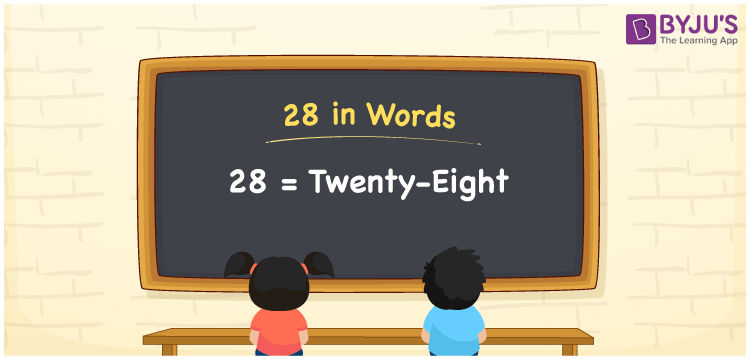# 28 in Words

We can write 28 in words as Twenty-eight. Conversion of the number 28 into words is very simple since it is a two-digit number. If you have 28 fiction books, then you can say, “I have Twenty-eight fiction books with me”. In this article, you will learn how to write the cardinal number 28 in words.

 28 in words Twenty-eight Twenty-eight in Numbers 28

## 28 in English Words

We generally write numbers in words using the English alphabet. Thus, we can read 28 using the words in English as “Twenty-eight”.## How to Write 28 in Words?

We know that 28 is a two-digit number so let’s make a place value chart with two columns as shown below.

 Tens Ones 2 8

Here, ones = 8 and tens = 2.

This can be combined as given below.

2 × Tens + 8 × Ones

= 2 × 10 + 8 × 1

= 20 + 8

= 28

= Twenty-eight

Therefore, 28 in words = Twenty-eight.

### Facts About the Number 28

28 is a natural number that is the successor of 27 and the predecessor of 29.

28 in words – Twenty-eight

Is 28 an odd number? – No

Is 28 an even number? – Yes

Is 28 a prime number? – No

Is 28 a composite number? – Yes

Is 28 a perfect square number? – No

Is 28 a perfect cube number? – No

How do you write 28 in Roman numerals? – XXVIII

## Frequently Asked Questions on 28 in Words

Q1

### How can I write 28 in words?

The spelling of 28 in words is Twenty-eight, which means you can express 28 in words as Twenty-eight.
Q2

### How do you spell 10^28 in words?

10^28 can be spelt as “Ten to the power twenty-eight”.
Q3

### What is the value of six plus twenty-eight?

Six plus twenty-eight = 6 + 28 = 34 Thus, the value of six plus twenty-eight is thirty-four.